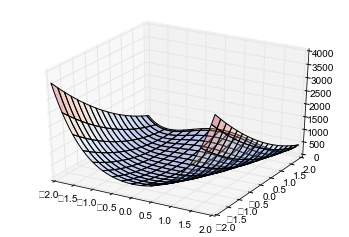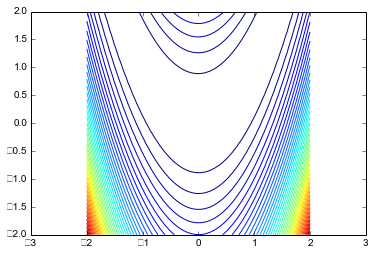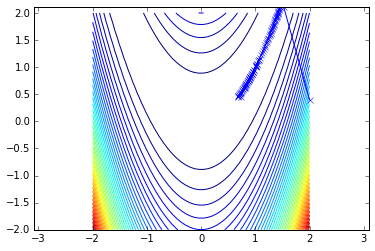# Newton's method in $n$ dimensions

In :
import numpy as np
import numpy.linalg as la

import scipy.optimize as sopt

import matplotlib.pyplot as pt
from mpl_toolkits.mplot3d import axes3d


Here are two functions. The first one is an oblong "bowl-shaped" one made of quadratic functions.

In :
def f(x):
return 0.5*x**2 + 2.5*x**2

def df(x):
return np.array([x, 5*x])

def ddf(x):
return np.array([
[1,0],
[0,5]
])


The second one is a challenge problem for optimization algorithms known as Rosenbrock's banana function.

In :
def f(X):
x = X
y = X
val = 100.0 * (y - x**2)**2 + (1.0 - x)**2
return val

def df(X):
x = X
y = X
val1 = 400.0 * (y - x**2) * x - 2 * x
val2 = 200.0 * (y - x**2)
return np.array([val1, val2])

def ddf(X):
x = X
y = X
val11 = 400.0 * (y - x**2) - 800.0 * x**2 - 2
val12 = 400.0
val21 = -400.0 * x
val22 = 200.0
return np.array([[val11, val12], [val21, val22]])


Let's take a look at these functions. First in 3D:

In :
fig = pt.figure()
ax = fig.gca(projection="3d")

xmesh, ymesh = np.mgrid[-2:2:50j,-2:2:50j]
fmesh = f(np.array([xmesh, ymesh]))
ax.plot_surface(xmesh, ymesh, fmesh,
alpha=0.3, cmap=pt.cm.coolwarm, rstride=3, cstride=3)

Out:
<mpl_toolkits.mplot3d.art3d.Poly3DCollection at 0x7ff479b7de10>

/usr/lib/python3/dist-packages/matplotlib/backends/backend_agg.py:517: DeprecationWarning: npy_PyFile_Dup is deprecated, use npy_PyFile_Dup2
filename_or_obj, self.figure.dpi)Then as a "contour plot":

In :
pt.axis("equal")
pt.contour(xmesh, ymesh, fmesh, 50)

Out:
<matplotlib.contour.QuadContourSet at 0x7ff4a4a897f0>• You may need to add contours to seee more detail.
• The function is not symmetric about the y axis!

### Newton

First, initialize:

In :
# Initialize the method

guesses = [np.array([2, 2./5])]


Then evaluate this cell lots of times:

In :
x = guesses[-1]
s = la.solve(ddf(x), df(x))
next_guess = x - s
print(f(next_guess), next_guess)

guesses.append(next_guess)

0.00468110908297 [ 0.93196634  0.86783648]



Here's some plotting code to see what's going on:

In :
pt.axis("equal")
pt.contour(xmesh, ymesh, fmesh, 50)
it_array = np.array(guesses)
pt.plot(it_array.T, it_array.T, "x-")

Out:
[<matplotlib.lines.Line2D at 0x7f0f62b645c0>]